# Magnitude Of Acceleration: 5 Facts You Should Know

Acceleration is the rate at which velocity changes. Because velocity is a vector quantity, acceleration is also a vector quantity. As a result, it requires magnitude as well as direction. So we are going to look at how to find magnitude of acceleration in this article.

We utilise formulae and relations to calculate magnitude. We can represent magnitude in terms of units. As acceleration is defined as a change in velocity with time, the SI unit for it is . Acceleration is affected by various factors such as velocity, time, force, and so on. We’ll go through a few different methods for estimating the magnitude of acceleration. Let’s read on.

## 1. How To Find Magnitude Of Acceleration From the definition of acceleration:

As we all know, acceleration refers to the rate at which velocity changes. If a body’s initial velocity is vi and its final velocity is vf, the acceleration can be calculated by dividing the velocity change by the time interval Δt:

(Here, the vector quantity is shown in bold characters, and vertical lines stand for the magnitude of the vector or we can say the absolute value of the vector, which is always positive.)

Using velocity and time, the above equation may be used to determine the magnitude of acceleration.

## 2. How To Find Magnitude Of Acceleration From Newton’s second law:

Newton’s second law states that force is obtained by multiplying acceleration by the mass of the body. So, how can you figure out what the magnitude of the acceleration is?

So, according to Newton’s second law, force on a body is proportional to its acceleration, whereas mass is inversely proportional to acceleration. Let us translate these statements into the magnitude of acceleration formula: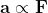and

Thus,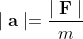## 3. How to Find Magnitude of Acceleration From vector components of acceleration:

Acceleration is a vector quantity, as we all know. To obtain this quantity, add the components of acceleration together. The simple rule of vector addition can be used here.If two vector components are involved, we can write:

In the Cartesian plane, we can utilise X and Y coordinates. In this circumstance, how do you determine the magnitude of acceleration? In a Cartesian coordinate system, the X and Y components are perpendicular to each other. The magnitude of the acceleration may be calculated by squaring the values and then calculating the square root of the sum.

As a result, the equation is as follows:

The magnitude of acceleration formula in three-dimensional space is:

## 4. How to Find Magnitude of Centripetal acceleration:

Because of the continuous change in direction in a circular motion, the velocity varies, resulting in acceleration.The acceleration is heading in the direction of the circle’s centre. Squaring the speed of the body v and dividing it by the distance of the body from the circle’s centre gives the magnitude of the centripetal acceleration. Thus, centripetal acceleration:

## 5. How To Find Magnitude Of Acceleration From equations of motion:

Equations of motion are essentially equations that explain the motion of any physical system and demonstrate the relationship between object displacement, velocity, acceleration, and time.

When the magnitude of the acceleration is constant, the kinematics equation of motion in one dimension is also used to calculate the magnitude of acceleration.

The following are the equations of motion:

•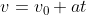•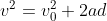•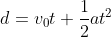When we make acceleration the subject of an equation, we obtain the following:

•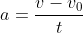(This is the same which we got from the definition of acceleration.)
•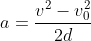•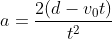This is how we can find the magnitude of acceleration.

Solved Examples On finding Magnitude of Acceleration:

Problem 1:

## A car starts from rest and achieves a speed of 54 km/h in 3 seconds. Find its acceleration?

Solution: A car starts from rest. Thus, initial velocity of a car

Vi  = 0 m/s

Vf  = 54 km/h

= (54 ✕ 1000) / 3600

= 15 m/s

Δt = 3 s

Thus, Acceleration : a = (Vf – Vi) / Δt

= (15 – 0) / 3

=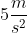Problem 2:

## Determine the accelerations that result when a 12 N net force is applied to a 3 kg object and then to a 6 kg object.

Solution: Force applied F = 12 N

Masses of object m1 = 3 kg

m2 = 6 kg

Acceleration of object having mass 3 kg

a1 = F / m1

= 12 / 3

=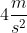Acceleration of object having mass 6 kg

a2 = F / m2

= 12 / 6

=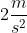Because mass and acceleration are inversely proportional, we can observe that as mass rises, acceleration decreases.

Problem 3:

## , where x is in meters and t is in seconds. Find the acceleration of the body when t = 3 s.

Solution: Here :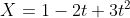t  = 3s

Velocity v = dX/dt

= d/dt ()

= -2 + 6t

Acceleration : a = dv/dt

= d/dt (-2 + 6t)

=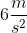As we can see, for this motion, acceleration is not time dependent; acceleration will be constant throughout the motion, and the magnitude of acceleration will be .

Problem 4:

## Calculate the centripetal acceleration of a point 7.50 cm from the axis of an ultra centrifuge spinning at  revolutions per minute.

Solution: Here we are given:

Distance from centre r = 7.5 cm

= 0.0750 m

Angular velocity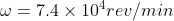Now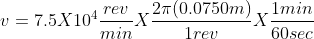= 589 m/s

Thus, centripetal acceleration: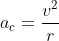=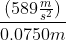=Alpa P. Rajai

I am Alpa Rajai, Completed my Masters in science with specialization in Physics. I am very enthusiastic about Writing about my understanding towards Advanced science. I assure that my words and methods will help readers to understand their doubts and clear what they are looking for. Apart from Physics, I am a trained Kathak Dancer and also I write my feeling in the form of poetry sometimes. I keep on updating myself in Physics and whatever I understand I simplify the same and keep it straight to the point so that it deliver clearly to the readers. You can also reach me at : https://www.linkedin.com/in/alpa-rajai-858077202/